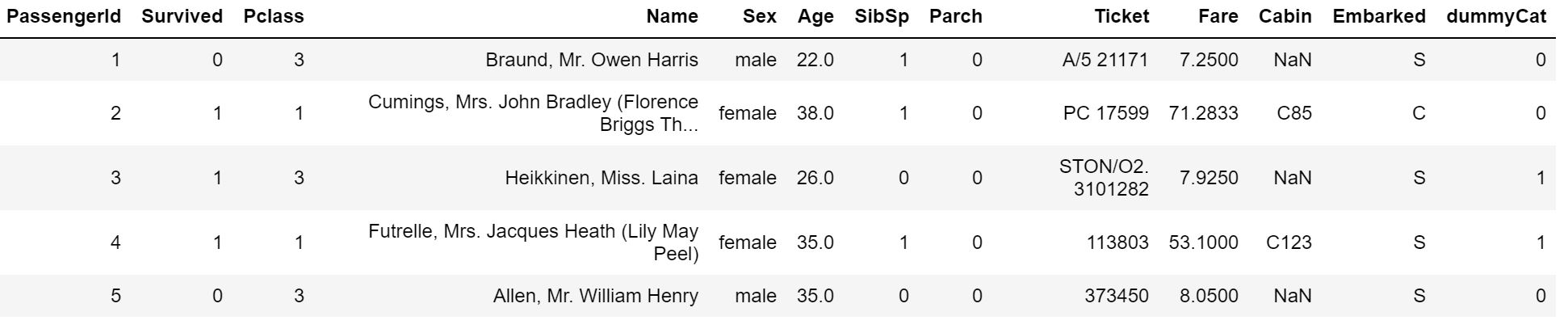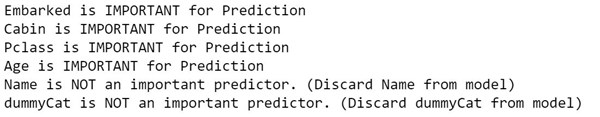Chi Square Feature Selection in Python

Introduction

Feature selection is an important part of building machine learning models. As the saying goes, garbage in garbage out. Training your algorithms with irrelevant features will affect the performance of your model. Also known as variable selection or attribute selection, choosing or engineering new features is often what separates the best performing models from the rest.

Features selection can be both an art and science and it’s a very broad topic. In this blog we will focus on one of the methods you can use to identify the relevant features for your machine learning algorithm and implementing it in python using the scipy library. We will be using the chi square test of independence to identify the important features in the titanic dataset.

After reading this blog post you will be able to:

• Gain an understanding of the chi-square test of independence
• Implement the chi-square test in python using scipy
• Utilize the chi-square test for feature selectionGetting Started

To get started, we need a dataset to play with. We will be using the famous Titanic Dataset through this post. I am sure you have heard of the Titanic. The famous largest passenger ship of its time that collided with an iceberg on April 15, 1912. Many people lost their lives in this tragedy that caused shock to the international community. One of the reasons for such a tragedy was that there were not enough lifeboats. Many lucky people did survive. Can we use machine learning to predict who would survive? Of course, we can! Among the first steps you would need to do is identify the important features to use in your machine learning model. This is the focus of this post.

The titanic dataset contains data for 887 of the real Titanic passengers with an attribute of Survived that determines whether the person survived or not. Aside from this, there are other attributes such as Sex, Age, the Fare paid, Pclass, Name among others.

You can download the titanic dataset from:

Chi Square Test

The Chi-Square test of independence is a statistical test to determine if there is a significant relationship between 2 categorical variables.  In simple words, the Chi-Square statistic will test whether there is a significant difference in the observed vs the expected frequencies of both variables.

The Chi-Square statistic is calculated as follows:

The Null hypothesis is that there is NO association between both variables.

The Alternate hypothesis says there is evidence to suggest there is an association between the two variables.

In our case, we will use the Chi-Square test to find which variables have an association with the Survived variable. If we reject the null hypothesis, it's an important variable to use in your model.

To reject the null hypothesis, the calculated P-Value needs to be below a defined threshold. Say, if we use an alpha of .05, if the p-value < 0.05 we reject the null hypothesis. If that’s the case, you should consider using the variable in your model.

Rules to use the Chi-Square Test:

1. Variables are Categorical

2. Frequency is at least 5

3. Variables are sampled independently

Chi-Square Test in Python

We will now be implementing this test in an easy to use python class we will call ChiSquare. Our class initialization requires a panda’s data frame which will contain the dataset to be used for testing. The Chi-Square test provides important variables such as the P-Value mentioned previously, the Chi-Square statistic and the degrees of freedom. Luckily you won’t have to implement the show functions as we will use the scipy implementation for this.

Let’s begin creating our class.

import pandas as pd
import numpy as np
import scipy.stats as stats
from scipy.stats import chi2_contingency

class ChiSquare:
def __init__(self, dataframe):
self.df = dataframe
self.p = None #P-Value
self.chi2 = None #Chi Test Statistic
self.dof = None

self.dfTabular = None
self.dfExpected = None

Next, we define our function called _print_chisquare_result which will accept as an input the name of a column X and the alpha value. If you remember, alpha is the threshold that will be used to determine if to reject or accept the null hypothesis of the Chi-Square test of independence. This function will print if the variable X is important or if not. If you look at the code, it’s comparing the p-value (which we will implement next) against this threshold.

An easy to use way to remember this logic of accepting or rejecting the null hypothesis is the following quote:

If P is low, Ho (null hypothesis) must go...
def _print_chisquare_result(self, colX, alpha):
result = ""
if self.p<alpha:
result="{0} is IMPORTANT for Prediction".format(colX)
else:
result="{0} is NOT an important predictor. (Discard {0} from model)".format(colX)

print(result)

Now we implement the actual logic to performing the Chi-Square test using scipy in our new function called TestIndependence. This function accepts two column names, colX and colY we are the two variables being compared. When using this class, colY is your objective, the variable you are trying to predict, Survived in our titanic dataset. ColX is the feature you are testing against. The last variable is Alpha which we default to 0.05.

First we convert our colX and colY to string types. Remember the Chi-Square test requires categorical variables.

To calculate our frequency counts we will be using the pandas crosstab function. The observed and expected frequencies will be stored in the dfObserved and dfExpected dataframes as they are calculated.

Finally, we use the scipy function chi2_contingency to calculate the Chi-Statistic, P-Value, Degrees of Freedom and the expected frequencies. One line for all the functions mentioned in the chi-square test section! We then simply store them in our class variables.

The last step is we call the _print_chisquare_result that performs the logic previously defined and tells the result of the test for our feature selection.

def TestIndependence(self,colX,colY, alpha=0.05):
X = self.df[colX].astype(str)
Y = self.df[colY].astype(str)

self.dfObserved = pd.crosstab(Y,X)
chi2, p, dof, expected = stats.chi2_contingency(self.dfObserved.values)
self.p = p
self.chi2 = chi2
self.dof = dof

self.dfExpected = pd.DataFrame(expected, columns=self.dfObserved.columns, index = self.dfObserved.index)

self._print_chisquare_result(colX, alpha)

Chi-Square Feature Selection in Python

We are now ready to use the Chi-Square test for feature selection using our ChiSquare class. Let’s now import the titanic dataset. The second line below adds a dummy variable using numpy that we will use for testing if our ChiSquare class can determine this variable is not important. This dummy variable has equal chances of being a 1 or 0 in each row.

df['dummyCat'] = np.random.choice([0, 1], size=(len(df),), p=[0.5, 0.5])

The variable df is now a pandas dataframe with the below information:Almost there!

Let’s now initialize our ChiSquare class and we will loop through multiply columns to run the chi-square test for each of them against our Survived variable. The class then prints if the feature is an important feature for your machine learning model. You’ll notice among the not important ones is our dummyCat variable.

#Initialize ChiSquare Class
cT = ChiSquare(df)

#Feature Selection
testColumns = ['Embarked','Cabin','Pclass','Age','Name','dummyCat']
for var in testColumns:
cT.TestIndependence(colX=var,colY="Survived" )Conclusion

We went through a quick overview of the Chi-Square test and how it can be used for feature selection, implemented it in an easy to use class utilizing the scipy library to do the heavy lifting in performing the calculations and utilized pandas to calculate our frequencies table. Lastly, we used our Chi-Square class to perform a quick feature selection against the titanic dataset determining which variables would be helpful to our machine learning model. Keep in mind to not only rely on this test for feature selection. Other factors should be taken into consideration as feature selection is a broad topic.

Full Code

import pandas as pd
import numpy as np
import scipy.stats as stats
from scipy.stats import chi2_contingency

class ChiSquare:
def __init__(self, dataframe):
self.df = dataframe
self.p = None #P-Value
self.chi2 = None #Chi Test Statistic
self.dof = None

self.dfObserved = None
self.dfExpected = None

def _print_chisquare_result(self, colX, alpha):
result = ""
if self.p<alpha:
result="{0} is IMPORTANT for Prediction".format(colX)
else:
result="{0} is NOT an important predictor. (Discard {0} from model)".format(colX)

print(result)

def TestIndependence(self,colX,colY, alpha=0.05):
X = self.df[colX].astype(str)
Y = self.df[colY].astype(str)

self.dfObserved = pd.crosstab(Y,X)
chi2, p, dof, expected = stats.chi2_contingency(self.dfObserved.values)
self.p = p
self.chi2 = chi2
self.dof = dof

self.dfExpected = pd.DataFrame(expected, columns=self.dfObserved.columns, index = self.dfObserved.index)

self._print_chisquare_result(colX,alpha)